# What is Box plot and the condition of outliers?

Box plot is a data visualization plotting function. It shows the min, max, median, first quartile, and third quartile. All of the things will be explained briefly. All of the property of box plot can be accessed by `dataframe.column_name.describe()` function.

## Aspects of a box plot

Here is a well distributed data-set.

 `data ``=` `[``0``, ``1``, ``2``, ``3``, ``4``, ``5``, ``6``]  ` `df ``=` `pd.DataFrame(data, columns ``=` `[``'Num'``])   ` `df `

Output: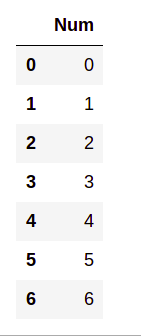Now plotting the data frame using box plot,

 `plt.figure(figsize ``=` `(``10``, ``7``))  ` ` `  `df.boxplot()  `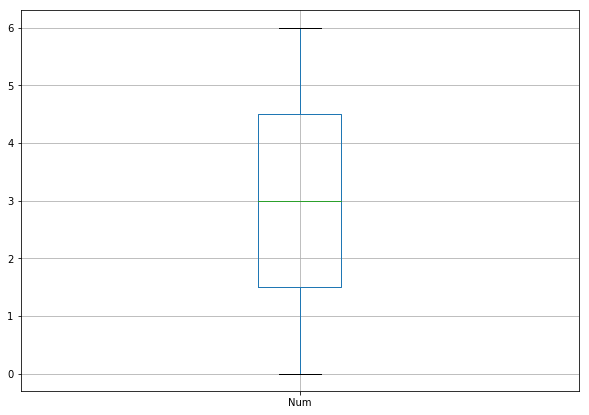#### Explanation of the different parts of the box plot

The maximum and the minimum is the max and min value of the data-set. 50 percentile is the median of the data-set. The first quartile is the median of the data between the min to 50% and the third quartile is the median of the data between 50% to max. The outliers will be the values that are out of the (1.5*interquartile range) from the 25 or 75 percentile.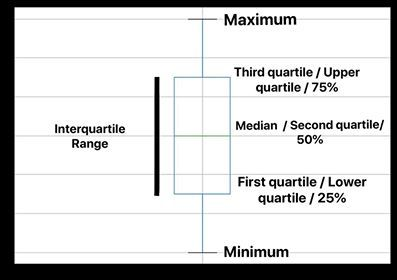### Methods of finding the values

• Use the median to divide the ordered data set into two halves.
1) If there is an odd number of data points in the original ordered data set, do not include the median (the central value in the ordered list) in either half.
2) If there is an even number of data points in the original ordered data set, split this data set exactly in half.

• The lower quartile value is the median of the lower half of the data. The upper quartile value is the median of the upper half of the data.
• An extreme value is considered to be an outlier if it is at least 1.5 interquartile ranges below the first quartile, or at least 1.5 interquartile ranges above the third quartile.

## Different Cases of Box Plot

Let us see different cases of box plots with different examples and let’s try to understand each one of them.

• `Now for the data = [0, 1, 2, 3, 6, 6, 6]`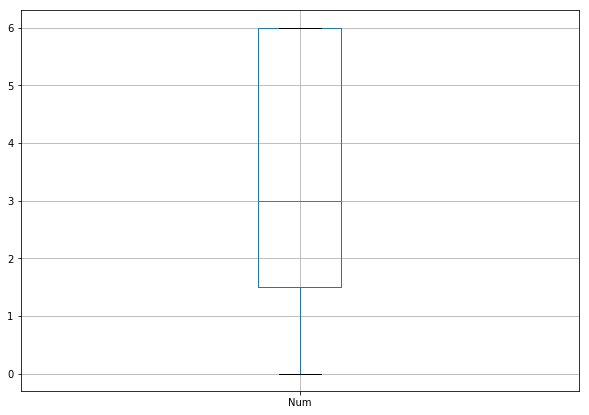Here the median of the data is 3, min is 0 and max is 6. The first quartile is 1.5 but after 50% to max values, all of the data is 6. So the third quartile and the max values are the same.

• `For the data = [0, 1, 2, 3, 4, 5, 9] `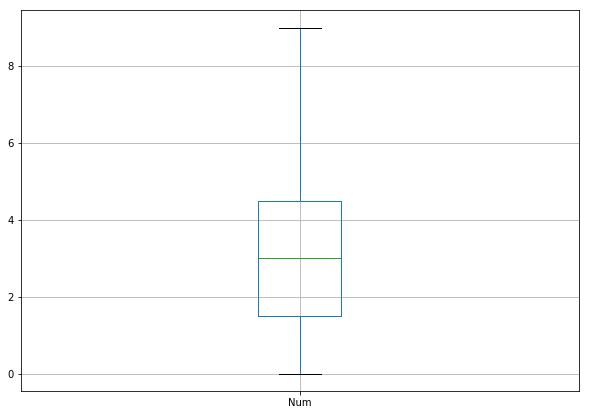Here the median is 3. For the third quartile, the values are 4, 5 and 9. So the third quartile is 5 and the max value is 9.

• `For the data = [0, 1, 2, 3, 4, 5, 10]`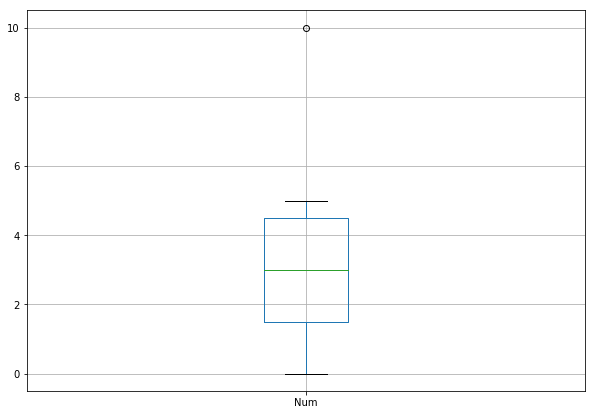Unlike the previous one, the max value is 5 because the third quartile is 4.5 and the interquartile range is `(4.5-1.5)=>3`. So, `1.5*3` is `4.5` and third quartile`(4.5)+4.5=>9`. So 10 is larger than the limit 9, thus it becomes an outlier.

## Description

The box plot seem useful to detect outliers but it has several other uses too. Box plots take up less space and are therefore particularly useful for comparing distributions between several groups or sets of data. It is a direct representation of the Probability Density Function which indicates the distribution of data.

My Personal Notes arrow_drop_upCheck out this Author's contributed articles.

If you like GeeksforGeeks and would like to contribute, you can also write an article using contribute.geeksforgeeks.org or mail your article to contribute@geeksforgeeks.org. See your article appearing on the GeeksforGeeks main page and help other Geeks.

Please Improve this article if you find anything incorrect by clicking on the "Improve Article" button below.